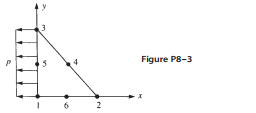### Create an Account

Home / Questions / Express the strains ex ey and gxy for the element of Figure 8 3 by using the results given...

# Express the strains ex ey and gxy for the element of Figure 8 3 by using the results given in Section 8.2 Evaluate these strains at the centroid of the element then evaluate the stresses at the

Express the strains ex;ey, and gxy for the element of Figure 8–3 by using the results given in Section 8.2. Evaluate these strains at the centroid of the element; then evaluate the stresses at the centroid in terms of E and n. Assume plane stress conditions apply.Aug 01 2020 View more View LessSubscribe To Get Solution